Published: 06 October 2023

# Application of optimized CNN algorithm in landslide boundary detection

Lili Wang1
Yun Qiao2
1, 2Department of Information Engineering, Youth College of Political Science of Inner Mongolia Normal University, Hohhot, China
Corresponding Author:
Lili Wang
Article in Press
Views 32

#### Abstract

Landslide, as a natural geological phenomenon with great harm, seriously threatens human social activities and life safety. It has a variety of latent and immeasurable destructiveness, which has a significant impact on the economic losses in rural areas. Therefore, it is urgent to take measures to accurately identify landslides to reduce their negative impacts. However, traditional manual visual interpretation has been unable to meet the current needs for emergency rescue of landslides, so computer intelligent methods have been paid attention to. This study proposes a new recognition network to address the problem of low accuracy of intelligent landslide boundary recognition methods. Firstly, the experiment incorporated boundary structure information into the Full Convolutional Network (FCN) for optimization, and constructed an Improved Full Convolutional Network (IFCN) model to better achieve image reconstruction. After that, Attention Mechanism (AM) is further introduced to achieve accurate detection of landslide boundaries in images, namely the IFCN-AM model. The attention mechanism introduced include spatial attention mechanism and multi-channel attention mechanism. Both are responsible for enhancing the language representation ability of the model and aggregating the interrelated features between different channels. The experimental results show that IFCN-AM has a 3 % to 7 % improvement in accuracy, recall, F1 value, and MIoU value.

## 1. Introduction

Landslide is a serious natural geological phenomenon. This phenomenon is mainly affected by natural geological processes and human activities. The rock and soil on the slope slide downward under the action of gravity . China is a vast country with relatively complex geological conditions and geomorphological forms, so landslides are also very common. However, the destructive nature of landslides is difficult to measure. Landslides in rural areas are highly likely to completely destroy public facilities such as farmland, houses, and highways, causing significant economic losses. In addition, the sliding of mountain landslides is slow and long-term, or intermittent . It can be delayed for several years, decades, or even hundreds of years, and its scale can reach millions of cubic meters, posing a serious threat to human and biological life safety. Therefore, it is urgent to take relevant measures to accurately identify landslides in order to reduce the impact of landslide disasters . How to quickly extract landslide information to meet disaster emergency response is a key research point. The rapid development of remote sensing technology in recent years has greatly improved traditional geological hazard monitoring methods and provided more direct data support for geological hazard evaluation . Traditional landslide boundary recognition methods mainly rely on manual visual interpretation, but they cannot meet the current needs of emergency rescue. In this context, by using remote sensing images, there is a relatively complete theoretical basis for applying computer intelligence technology to extract targets’ boundary features . However, the existing methods do not involve a deeper network structure, and it needs improve image recognition accuracy. In view of this, to improve landslide boundary recognition accuracy, this study incorporated images’ boundary structure information into the Full Convolutional Neural Network (FCN) and constructed an IFCN network. On this basis, a spatial and multi-channel dual attention mechanism (AM) is introduced to achieve accurate detection of landslide boundaries in images.

## 2. Related work

Convolutional Neural Network (CNN) is an intelligent algorithm incurrent scientific research field, which has strong applicability in various object detection. Therefore, the current research on CNN detection performance has also yielded many achievements. Sambolek and Ivasic-Kos proposed a probe search and rescue model based on Fast Region Convolutional Neural Network based on mountain exploration tourism search and rescue operations. The detector can detect the injured and can be applied to search and rescue operations in abnormal areas covered by vegetation. A dataset simulating rescue scenarios was developed to verify model’s effectiveness. The final model achieved excellent results in search and rescue scenarios . Liu and other scholars discussed food detection and proposed a CNN based food feature extraction model. The model can detect food features such as meat, aquatic products, grains, fruits, vegetables, and so on through complex food matrices. The simulation results show that the combination of CNN and non-destructive testing technology has great potential for detecting and analyzing complex food substrates . Saponara S et al. utilized a fire monitoring system combining YOLOv2 and CNN to achieve real-time detection of fire and smoke. The overall system uses lightweight neural networks to extract features from fire and smoke images. After testing, this system has better detection effects compared to other methods, and is suitable for intelligent real-time fire detection and monitoring systems . Based on Region Convolutional Neural Network, an object detection method is proposed by Wu et al. from object detection topic in the field of computer vision. Experiments have optimized Region Convolutional Neural Network by proposing a mask Region Convolutional Neural Network model. The intersection point of this model is higher than the threshold, making it easy to obtain high-quality samples, enabling the detection of small-scale works. The validation results on the dataset demonstrate the superior detection performance of Mask Region Convolutional Neural Network . From the perspective of public safety, Ullah team proposed a pedestrian abnormal behavior detection model that combines multi-layer bidirectional short-term memory algorithm and CNN. This model can extract the depth characteristics of pedestrians and achieve the detection and capture of abnormal events in smart city monitoring scenarios. The report results on the dataset show that the proposed model can improve the detection accuracy by 41.8 % .

Landslide detection technology has been a hot topic for researchers for a long time, and there are many research achievements at present. Gao and other researchers proposed a framework for landslide recognition based on semantic segmentation networks for landslide recognition and mapping. The framework utilizes a bottleneck structure to control parameters, and utilizes an encoder decoder structure to improve the testing speed of the model. In addition, the experiment also constructed a dataset to enrich the data used for model training. The constructed framework improves the efficiency of landslide boundary detection and exhibits great application potential . Shi and other scholars proposed a landslide boundary detection technology that combines machine learning algorithms and remote sensing images. This technology realizes the recognition of mountain landslides through feature extraction methods such as tracking extraction, source point extraction, and attribute extraction, and can be applied to landslide boundary detection with complex land cover. The proposed technology also uses image processing and parallel block processing strategies to improve detection speed. The practical application results show that the proposed technology can achieve a recognition accuracy rate of over 80 % and is practical . Scientific researchers such as Ji et al. use high-resolution optical satellite images to detect landslide boundaries. In order to cope with the diversity and complexity of latent landslides, the holistic model introduces an attention mechanism to achieve more accurate landslide feature extraction. In order to verify the effectiveness of the proposed method, a mountain landslide dataset was constructed experimentally. Theproposed method achieves a detection score of 96.62 % for landslide boundaries, with high advantages . Yao et al. constructed detection techniques using neural networks and remote sensing images from the perspective of detecting objects with blurred landslide boundaries. The experiment takes the boundary detection of a loess landslide as an example to detect its blurred boundary objects. By comparing the detection performance of remote sensing images and visual images, it is found that this study can provide reference value for the detection of fuzzy objects at the landslide boundary . Hacıefendio et al. in order to determine the impact area of the landslide boundary, the Lu K. team used visualization technology to assess the possibility of landslides occurring in local areas. In the experiment, four types of models were selected for automatic monitoring of regional landslides, and comparative verification was conducted. The receiver working curve and f50 scoring results of landslide detection characteristics show that visualization technology can effectively detect landslide zones and has reliability .

In summary, some scholars have now made improvements to CNN to adapt to their research fields. At the same time, landslide detection technology has also received considerable attention in recent years. However, there is no deeper structure in the current network structure, and the accuracy of landslide boundary recognition needs to be improved. Therefore, this study constructs an Improved Full Convolutional Network based on the boundary structure information of Full Convolutional Network fused images. And a dual attention mechanism of spatial and multi-channel is introduced to achieve accurate detection of landslide boundaries in the image.

## 3. Landslide boundary detection model based on full convolutional neural network and attention mechanism

### 3.1. Construction and improvement of full convolutional network based boundary detection model

CNN is a deep learning model that primarily classifies and recognizes images using matrix principles in linear algebra. Its basic structure is a convolutional layer, a pooling layer, and a fully connected layer, which are respectively responsible for image feature extraction, reducing the amount of learning parameters, and synthesizing the extracted features. CNN has a significant effect on single label or few label classification issues, but it has a poor effect on image semantic segmentation tasks [16-17]. Therefore, Full Convolutional Network came into being. The difference from traditional CNN is that original fully-connected-layer is replaced by Full Convolutional Network to CNN’s last layer with convolutional layer. After the feature image is pooled, it is restored to input image size by using bilinear interpolation to calculate classification losses pixel by pixel and obtain segmentation results. In image detection, Full Convolutional Network can accept different kinds of images. In this method, a single convolution can be used to catch different kinds of image region features . The Full Convolutional Network model includes two parts: a full convolutional network and a loss layer. The former can express image features. The latter mainly undertakes calculation of image loss. So model parameters can be obtained and the former can be optimized. Fig. 1 shows the basic structure of an Full Convolutional Network.

Although Full Convolutional Network can achieve good detection performance for semantically segmented images, there are still some drawbacks. First, when extracting image depth features, Full Convolutional Network is difficult to obtain structural information such as image boundaries, which is not conducive to network back-end reconstruction and detection of images. Secondly, in some Full Convolutional Networks, it is necessary to connect two temporal phase diagrams in series, making the two temporal phase diagrams in the Full Convolutional Network a single temporal phase diagram, which is also detrimental to image reconstruction. Thirdly, when Full Convolutional Network performs change detection, it will introduce the original deep features of the front-end into the back-end, resulting in feature redundancy issues [19-20]. Therefore, in response to the above issues, this study further optimizes the Full Convolutional Network. That is, Full Convolutional Network incorporates image boundary structure information to form an Improved Full Convolutional Network model. Gaussian filter can make image noise reduced when detecting. The specific function is shown in Eq. (1):

1
$G\left(x,y\right)=\frac{1}{\sqrt{2\pi }\mu }\mathrm{e}\mathrm{x}\mathrm{p}\left(\frac{-\left({x}^{2}+{y}^{2}\right)}{2{\mu }^{2}}\right),$

where, $G\left(x,y\right)$ represents a Gaussian function; $\mu$ indicates the smoothing scale parameter; $\left(x,y\right)$ is the image coordinates entered. The smooth image obtained by convolving the input image with Gaussian function is shown in Eq. (2):

2
${f}_{s}\left(x,y\right)=f\left(x,y\right)*G\left(x,y\right),$

where, ${f}_{s}\left(x,y\right)$ represents a smooth image; $f\left(x,y\right)$ represents an input image; $\mathrm{*}$ represents a convolution operation. The gradient size of each pixel in the obtained smooth image is shown in Eq. (3):

3
$M\left(x,y\right)=\sqrt{{g}_{x}^{2}+{g}_{y}^{2}},$

where, ${g}_{x}$, ${g}_{y}$ are pixel points’ gradient operators. Each pixel’s direction in smoothed image is shown in Eq. (4):

4
$\alpha \left(x,y\right)=\mathrm{a}\mathrm{r}\mathrm{c}\mathrm{t}\mathrm{a}\mathrm{n}\left(\frac{{g}_{y}}{{g}_{x}}\right),$

where, ${g}_{x}$, ${g}_{y}$ are also a gradient operator for pixel points. The calculation equation for the gradient operator is shown in Eq. (5):

5
${g}_{x}=\frac{\partial {f}_{s}}{\partial x},{g}_{y}=\frac{\partial {f}_{s}}{\partial y}.$

Fig. 1Comparison of full convolutional network and CNN structures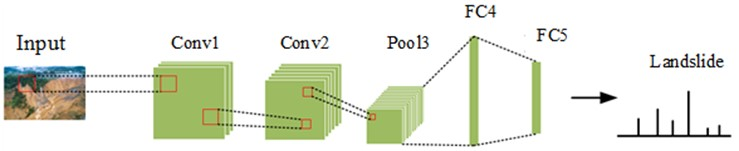a) CNN structure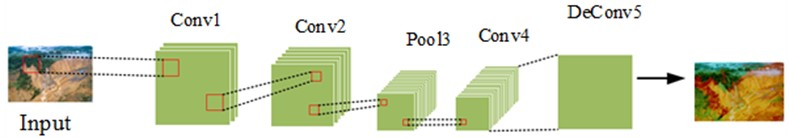b) FCN structure

From Eq. (5), it is not difficult to find that each gradient operator is the partial derivative for solving the coordinates of the smoothed image. After that, the center pixel in the neighborhood of each pixel 3×3 is compared with the pixel in the gradient direction. If central pixel is larger than gradient amplitude of two adjacent pixel points’ gray values along gradient direction, the point can be used as a boundary point. If the pixel point is greater than the set maximum threshold value, the point is the final boundary point. After that, subtract the resulting edge maps ${g}_{1}\left(x,y\right)$ and ${g}_{2}\left(x,y\right)$ to obtain a boundary difference map, as shown in Eq. (6):

6
${g}_{di}\left(x,y\right)={g}_{2}\left(x,y\right)-{g}_{1}\left(x,y\right),$

where, ${g}_{di}\left(x,y\right)$ represents a boundary difference diagram. In order to extract the depth features of the boundary difference map, the convolution kernel of $k×k$ was selected for the experiment. Assuming the convolutional layer is the $l$-th layer, the $j$ feature map of that layer is shown in Eq. (7):

7
${z}_{j}^{l}=g\left(\sum _{i\in {M}^{l-1}}{z}_{i}^{l-1}\mathrm{*}{w}_{ij}^{l}+{b}_{j}^{l}\right),$

where, ${z}_{j}^{l}$ represents the $j$-th characteristic diagram of the $l$-th layer; ${z}_{i}^{l-1}$ represents the $i$-th characteristic diagram of the $l-1$-th layer; ${w}_{ij}^{l}$ represents a convolution kernel from ${z}_{i}^{l-1}$ to ($A{z}_{j}^{l}$); ${b}_{j}^{l}$ is $j$-th feature map’s offset of $l$-th layer; $g\left(.\right)$ indicates the activation function; ${M}^{l-1}$ represents the number of feature maps for layer $l-1$. Assuming that the $m$-th layer is a pooling layer, the$j$-th feature map of the $m$-th layer is shown in Eq. (8):

8
${z}_{j}^{m}=g\left(\sum _{i\in {M}^{i-1}}{\beta }_{j}^{m}\cdot down\left({z}_{i}^{m-1}\right)+{b}_{j}^{l}\right),$

where, $g\left(.\right)$ represents the activation function; ${\beta }_{j}^{m}$ represents $j$-th characteristic graph’s pooling parameter of $m$-th layer; $down\left(\cdot \right)$ represents a pooling function; ${z}_{i}^{m-1}$ represents the $i$-th characteristic diagram of the $m-1$-th layer; ${b}_{j}^{m}$ represents the offset of the $j$-th feature map of the $m$-th layer. After performing convolution and pooling operations, the network has generated relatively rich global information. However, when performing feature recognition for landslides, the landslide area in the image is the key analysis object. If the analysis of landslide areas can be implemented, it will reduce the computational complexity of the network and improve its work efficiency. Therefore, based on the experimental proposal of fusing Full Convolutional Network with boundary image change information, AM is further introduced to achieve the detection of key information such as landslides in the image.

### 3.2. Construction of landslide boundary detection model combining attention mechanism and improved full convolutional network algorithms

In the process of landslide boundary detection, Full Convolutional Network will assign the same computational weight to all pixels in the overall feature map, which requires a long computational time [21-22]. However, the main object of this study is the landslide boundary, and calculating the overall feature map will reduce the operational efficiency of the algorithm. Therefore, in order to emphasize the key factor of landslide boundary, this experiment introduced attention mechanism, namely IFCN-AM model. attention mechanism can improve model’s detection performance by emphasizing foreground pixels and suppressing background pixels. IFCN-AM model can quickly scan global information to determine the key information and detection range to be focused on in the experiment . Subsequently, the extracted information flow is passed to the backend in a weighted order, while suppressing the flow of invalid information. This experiment introduces a combination of dual attention mechanism and Full Convolutional Network networks to better achieve images feature representation and improve landslide segmentation accuracy . The dual attention mechanism is divided into a spatial attention module and a multi-channel attention module. The spatial attention mechanism can establish the relationship between local features of an image, allowing extensive contextual information to be encoded and converted into local features to enhance the language representation ability of the model. Fig. 2 shows the structure of the spatial attention module. After inputting the landslide feature map, the correlation strength matrix between the two feature points obtained from the original feature after convolution and dimensionality reduction is first calculated through the model.

Fig. 2Spatial attention module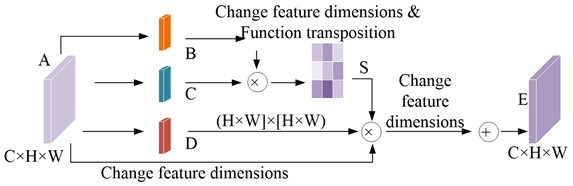The spatial attention map obtained after model normalization is shown in Eq. (9):

9
${S}_{ij}=\frac{\mathrm{e}\mathrm{x}\mathrm{p}\left({B}_{i}×{C}_{j}\right)}{{\sum }_{i=1}^{N}\mathrm{e}\mathrm{x}\mathrm{p}\left({B}_{i}×{C}_{j}\right)},$

where, ${B}_{i}$ is $i$-th pixel position in feature map $B$; ${C}_{j}$ stands for $j$-th pixel position in feature map $C$. ${S}_{ij}$ can evaluate $j$-th pixel position’s impact in the feature map on the $i$-th pixel position feature. At the same time, the original features are input into the convolution layer to generate a new feature map. Multiply the final result by the scale parameter, and perform an element by element summation operation on the original features. The final output result is shown in Eq. (10):

10
${E}_{j}=\alpha \sum _{i=1}^{N}\left({S}_{ij}{D}_{i}\right)+{A}_{j},$

where, $\alpha$ represents the scale parameter; ${D}_{i}$ represents a matrix after changing the feature dimension; ${A}_{j}$ represents the pixel at the $j$-th position in the original feature map. The model selectively aggregates context information based on spatial attention maps to ensure consistency in spatial semantics and highlight key detection areas to improve the segmentation accuracy of the final result . In addition, Improved Full Convolutional Network has not yet paid attention to the correlation of segmentation results between feature channel graphs, so this experiment also introduced a channel attention mechanism after combining spatial attention mechanism. The channel attention mechanism can aggregate interrelated features between different channels to improve feature representation. Fig. 3 shows the module structure of channel attention.

From Fig. 3, this channel attention module first reshapes the original features, and then multiplies the original feature matrix by its transposition matrix. After the normalization operation, the characteristic diagram between the final channels is obtained, as shown in Eq. (11):

11
${x}_{ji}=\frac{\mathrm{e}\mathrm{x}\mathrm{p}\left({A}_{i}×{A}_{j}\right)}{{\sum }_{i=1}^{C}\mathrm{e}\mathrm{x}\mathrm{p}\left({A}_{i}×{A}_{j}\right)},$

where, ${A}_{i}$ and ${A}_{j}$ represent the original characteristic diagrams of channels $i$ and $j$, respectively. The meaning of ${x}_{ji}$ is the impact of the $i$-th channel on the $j$-th channel. After that, the feature maps between each channel are weighted and fused to create a global association between them to obtain stronger semantic response features. The output diagram of the final channel attention mechanism is shown in Eq. (12):

12
${E}_{j}=\beta \sum _{i=1}^{C}\left({x}_{ji}{A}_{i}\right)+{A}_{j},$

where, $\beta$ represents the scale parameter; $C$ is the length of the feature map. The meaning of ${E}_{j}$ is the final feature of each channel, which is obtained by weighting all channel features with the original features. It can simulate the long-term semantic dependency between feature maps. This model combines spatial attention mechanism with channel attention mechanism to improve the feature representation of the overall image. The resulting IFCN-AM model is shown in Fig. 4.

Fig. 3Channel attention module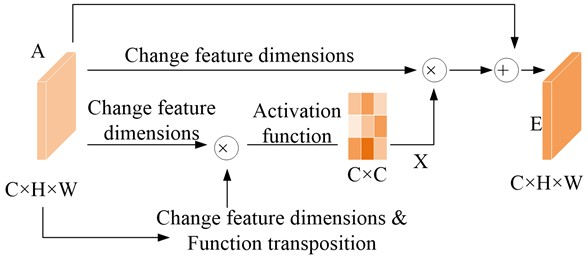Fig. 4IFCN-AM structure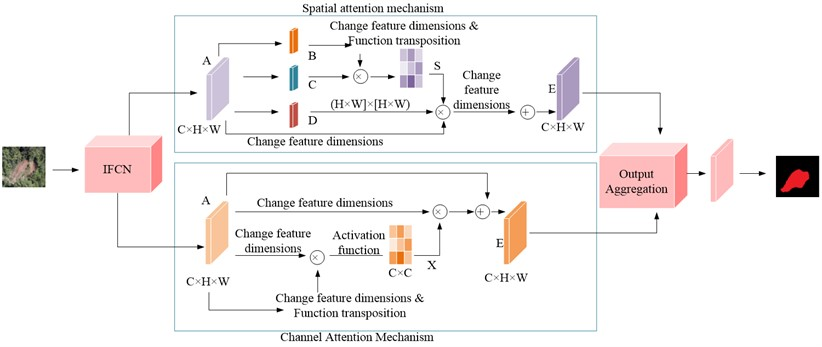To evaluate attention mechanism’s effect, this experiment uses Semantic Segmentation over Union (MIoU) as an evaluation index. Eq. (13) is the specific expression:

13
$MIoU=\frac{1}{k+1}\sum _{i=0}^{k}\left({p}_{ii}/\sum _{i=0}^{k}{p}_{ij}+\sum _{i=0}^{k}\left({p}_{ji}-{p}_{ii}\right)\right),$

where, $k$ represents categories classified number, which refers to landslide pixels and background pixels, so the value of $k$ is 1; ${p}_{ij}$ indicates a class $i$ pixel that has been misjudged as class $j$; ${p}_{ji}$ represents a class $j$ pixel that has been misjudged as a class $i$ pixel. Meanwhile, the experiment evaluates the performance of the model using recall rate, and its expression is shown in Eq. (14):

14
$Recall=\frac{TP}{TP+FN},$

where, $TP$ represents samples number whose positive class is predicted to be positive; $FN$indicates samples number whose positive class is predicted to be negative; $TN$ indicates samples number whose negative class is predicted to be negative. In addition, the experiment also selected the ${F}_{1}$ metric as an evaluation index to measure classification accuracy, and its expression is shown in Eq. (15):

15
${F}_{1}=2×\frac{Recall×Precision}{Recall+Precision},$

where, $Precision$ represents the accuracy rate of model detection.

## 4. Performance verification of landslide boundary detection model based on IFCN-AM

### 4.1. IFCN-AM model performance verification

The dataset used in this experiment collected a total of 2300 landslide image data, including three major categories. The first is to independently collect geological disaster image data in the Daqingshan region of Inner Mongolia, including various geological disaster image data such as landslides, debris flows, and collapses in the Daqingshan region of Inner Mongolia since 2003, and select landslide image data that can be used as model training. The landslide images in this section are mainly collected manually, and there are various types of landslides and complex backgrounds. The second is high-resolution landslide images taken using drones. This image collection has the characteristics of high image clarity, distinct characteristics of landslide mass, and easy determination of landslide mass boundaries. The third is the collected Public Remote Sensing Satellite Landslide Map, which has a surface resolution of 0.8 meters and is uniformly divided into 512×512 px size image. The landslide prediction model established based on a single data source can only obtain good discrimination ability for landslides from a certain source, while it is not ideal for other types of landslides. For example, the landslide discrimination model established solely using remote sensing data cannot achieve satisfactory prediction results in practical applications. Therefore, this experiment chooses to use this multi-source remote sensing image data to achieve accurate prediction of various types of landslide images, so as to meet more practical needs to a certain extent. For the selected landslide dataset, it is necessary to manually label the boundary and range of the landslide image to better train the model. Fig. 5 shows the final annotation result.

Fig. 6 shows the loss function curves of the Full Convolutional Network model, Improved Full Convolutional Network model, and IFCN-AM model on the training set and test set. Fig. 6(a) stands for loss function training curve. Fig. 6(b) stands for loss function test curve. As shown in Fig. 6(a), all three network models exhibit good training effects. After 100 iterations of the three models, the loss function curves tend to flatten out. As shown in Fig. 6(b), the IFCN-AM model has the best loss function curve on the test set, followed by Improved Full Convolutional Network. Therefore, introducing boundary change information and attention mechanism into the Full Convolutional Network model can enhance the generalization ability of the network.

Fig. 5Marking results of landslide datasets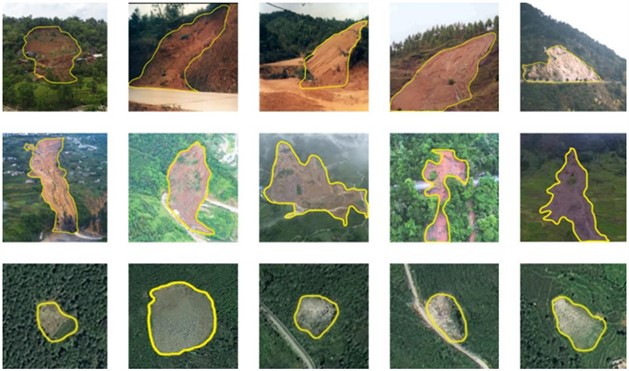Fig. 6Loss function curves for three models in training and test sets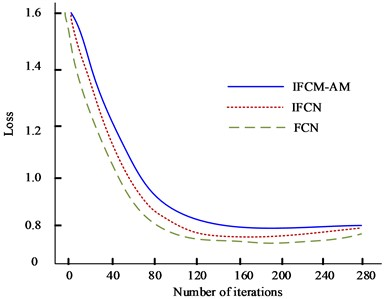a) Training set loss rate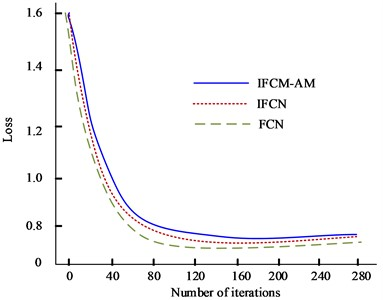b) Test set loss rate

Fig. 7 shows the training F1 curve of Full Convolutional Network model, Improved Full Convolutional Network model, and IFCN-AM model on training and test sets. Fig. 7(a) stands for F1 training curve, and Fig. 7(b) stands for F1 test curve. The F1 of the IFCN-AM model in the test set is higher than that of the other two networks, verifying the superior detection accuracy of the IFCN-AM model.

Fig. 7Training F1 curves for three models on training and test sets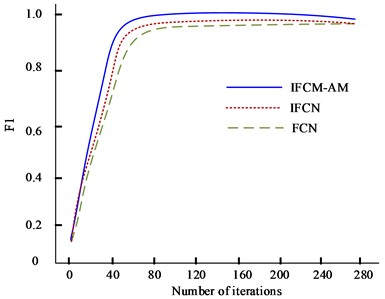a) Training set loss rate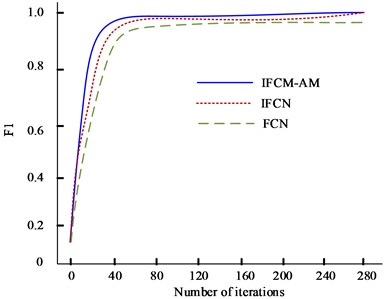b) Test set loss rate

In order to better compare the advantages of the IFCN-AM model, the current popular RCNN model, Faster-RCNN model, Improved Single Shot Multibox Detector (SSD) model, and You Only Look Once (YOLO) model were selected for the experiment. Fig. 8 stands for ROC curves for five models. The detection results of IFCN-attention mechanism model are closer to manual labeling results compared to the other five models. Therefore, the IFCN-AM model can improve the recall rate by 3 % to 4 % while reducing the requirements for error accuracy and time consumption.

Fig. 8PR curves for five models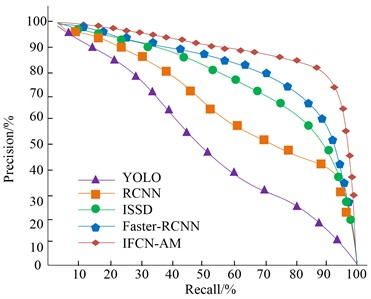Table 1 shows the comparative experimental results of landslide identification using five models. From this table, it is not difficult to find that compared to RCNN, Faster RCNN, Improved Single Shot Multibox Detector, and You Only Look Once, IFCN-AM has a 3 % to 7 % improvement in accuracy, recall, F1 value, and MIoU value. Therefore, the superiority of IFCN-AM in landslide detection is verified.

Table 1Comparison of experimental results of different models

 Model Precision Recall F1 MIoU Detection speed (Frame/s) RCNN 0.901 0.854 0.881 0.724 13.9 Faster-RCNN 0.919 0.887 0.903 0.789 11.2 ISSD 0.913 0.896 0.865 0.741 12.8 YOLO 0.906 0.881 0.845 0.732 13.6 IFCN-AM 0.978 0.913 0.926 0.801 11.1

### 4.2. Analysis of different types of landslide boundary detection results

According to the different material composition of landslides, they can be divided into soil landslides and rock landslides, with significant differences in the morphological characteristics of different landslides. To verify IFCN-AM model’s detection effect on different material composition types, the experiment then divided landslides into soil landslides and rock landslides based on whether there is vegetation coverage. 1300 pieces of data were selected for each landslide type to conduct experiments. Fig. 9 shows the training and validation curves for the selected five models. As can be seen from the figure, all five models have the ability to identify landslide boundaries. As the number of iterations increases, the IFCN-AM model exhibits optimal accuracy stability and low misclassification. This also verifies that incorporating the structural system information of the image boundary can effectively increase landslide boundary’s detailed information to improve model performance.

Table 2 shows the IFCN-AM identification results for different types of landslides. The indicators of the soil landslide and rock landslide selected in the experiment are basically the same. Comparing the vegetation coverage, the accuracy rate, recall rate, F1 value, and MIoU of landslides with vegetation coverage are higher than those of landslides without vegetation coverage. The calculation results show that IFCN-AM model has a good recognition effect for both soil and rock landslides.

Fig. 9Training and verification curves for five models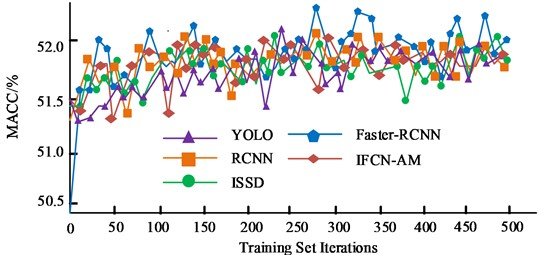a) Comparison of average accuracy of training sets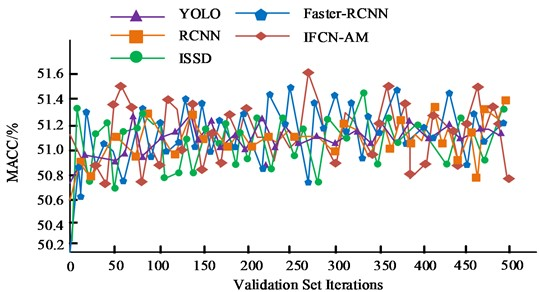b) Validation set average precision comparison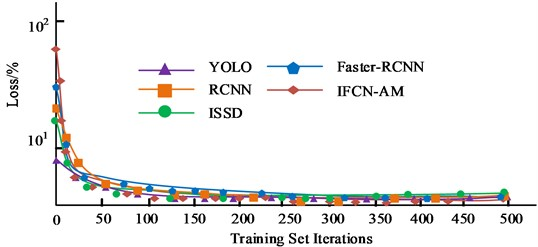c) Comparison of training set loss values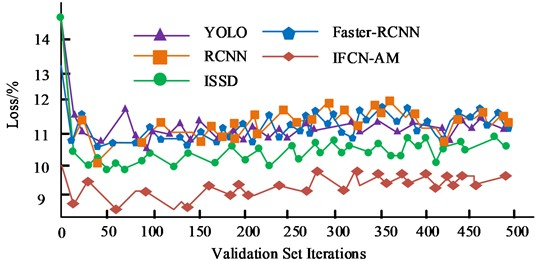d) Comparison of validation set loss values

Table 2Comparison of different types of landslide identification results

 Landslide type Precision Recall F1 MIoU Vegetation/soil landslide 0.971 0.925 0.917 0.813 No vegetation/soil landslide 0.982 0.889 0.889 0.798 Vegetation/rocky landslide 0.987 0.898 0.913 0.868 No vegetation/rocky landslide 0.969 0.883 0.899 0.754

Fig. 10 shows the final recognition effects of Full Convolutional Network, Improved Full Convolutional Network, and IFCN-AM on different landslides. As shown in the figure, the IFCN-AM model integrates the boundary structure information of the image and the dual attention mechanism, and the final recognized landslide boundary is the closest to the landslide boundary in the labeled map. Both the Full Convolutional Network and Improved Full Convolutional Network identification maps have a large number of misjudged areas, and the detailed boundary division of landslides is unclear. Therefore, IFCN-AM has certain advantages in identifying landslide boundaries.

Fig. 10Final recognition effects of full convolutional network, improved full convolutional network, and IFCN-AM on different landslides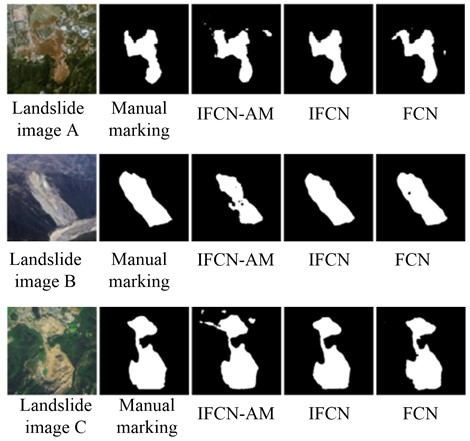## 5. Conclusions

Landslides have a variety of latent and immeasurable destructiveness, seriously threatening human social activities and life safety. In this study, a new recognition network, IFCN-AM model, was proposed to address the low accuracy of current intelligent landslide boundary recognition methods. The experiment selects landslide image data that can be used as model training from various geological disaster image data such as landslides, debris flows, and collapses in the Daqing Mountain region of Inner Mongolia from 2003 to now. The training and testing results of Full Convolutional Network model, Improved Full Convolutional Network model, and IFCN-AM model show that after 100 iterations, three models’ loss function curves tend to flatten out. IFCN-AM model has the highest F1 value. Compared to RCNN, Faster RCNN, ISSD, and YOLO, IFCN-AM has a 3 % to 7 % improvement in accuracy, recall, F1 value, and MIoU value. The detection results of different types of landslide boundaries show that the accuracy rate, recall rate, F1 value, and MIoU of vegetation covered landslides are higher than those of non vegetation covered landslides. The IFCN-AM model has good recognition effects for both soil and rock landslides. The IFCN-AM model integrates the boundary structure information of the image and the dual attention mechanism, and the final recognized landslide boundary is the closest to the landslide boundary in the labeled map, demonstrating certain advantages. This experiment basically achieves the experimental purpose. In the future, it can be considered to combine the landslide boundary detection system with the global positioning system to achieve the detection of large-scale landslide boundaries.

18 May 2023
Accepted
16 August 2023
Published
06 October 2023
Keywords
full convolutional network
spatial attention mechanism
channel attention mechanism
landslide boundary detection
deep learning
Acknowledgements

The research is supported by Scientific Research Project of Higher Education Institutions of Inner Mongolia Autonomous Region: Research on Geological Disaster Identification Technology of Daqing Mountain Based on Convolutional Neural Network (NJZY21579).

Data Availability

The datasets generated during and/or analyzed during the current study are available from the corresponding author on reasonable request.

Author Contributions

Lili Wang contributed to the motivation, the interpretation of the methods, the data analysis and results, and provided the draft versions and revised versions, references. Yun Qiao provided the data and results, the revised versions and references. All authors reviewed the manuscript.

Conflict of interest

The authors declare that they have no conflict of interest.